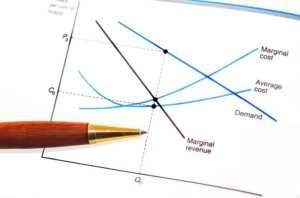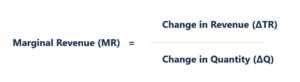# Marginal Revenue

Revenue obtained from selling an additional unit

## What is Marginal Revenue?

Marginal Revenue is the revenue that is gained from the sale of an additional unit. It is the revenue that a company can generate for each additional unit sold; there is a marginal cost attached to it, which has to be accounted for.

For example, Mr. A sells 50 packets of homemade chips every day and he incurs some cost to sell and produce them. He determined the price of each packet to be \$5, adding all the cost and his profit, where his profit is \$1.50 per packet. Now, Mr. A produced 55 packets one day by mistake and took all of them to the market. With no surprise, he was able to sell all 55 packets for \$5 each. He made his usual \$250 by selling 50 packets.

In addition to that, he sold 5 packets, which were produced by mistake. He was selling the packets for \$5 and since he sold 5 additional packets, he had a Marginal Revenue of \$25 (\$5 x 5). This is how Marginal Revenue is calculated. It is dependent on supply and demand, and on the type of market as well, such as Perfect Competition or Monopoly.In the image above, you can see three curves: Marginal Revenue, Average Revenue or Demand, and Marginal Cost.

### Marginal Revenue Curve

Let us examine the concept of Marginal Revenue in greater detail. The Marginal Cost curve is a “U”-shaped curve because the marginal cost for 1-5 additional units will be less, whereas with selling more incremental units, the marginal cost will begin to rise. The Marginal Revenue curve is sloping downwards because, with one additional unit sold, we would generate revenue close to our normal revenue but as we start selling more and more, we would be required to reduce the price of the item we are selling. Otherwise, we will not be able to sell all the units, which is also known as the law of diminishing margin. So, the more you sell after a normal limit, the more the price will diminish and, accordingly, so will revenue.

### Average Revenue Curve

There is an Average Revenue curve or Demand curve, which is not the consumers’ demand curve but rather the producers’ demand curve. The curve represents an average quantity at an average price. Now that we understand what these curves are and what their function is, let us discuss marginal revenue in the context of marginal cost.

Assume Mr. X is selling boxes of candy. He sells 25 boxes every day for \$2 each and makes a profit of \$0.50 on every box that he sells. Now, due to an increase in demand, he was able to sell 5 additional boxes of candy for the same price. He incurred the same cost, which leaves him with the same amount of profit on these boxes as well, which will add up to \$2.50 (\$0.50 x 5). Mr. X calculated that he could sell even more boxes of candy, so he ordered 10 more boxes. However, because of government restrictions and limited production, the cost of each box after the 30th box increased by 10%, which made the 5 additional boxes of candy cost \$1.65 each. His total cost = (30 boxes @ \$1.50 = \$45 and 5 boxes @ \$1.65 = \$8.25) total cost = \$45 + \$8.50 = \$53.50.

He went to the market and sold the candy boxes for the normal price of \$2 each for the first 30 boxes. After that, he priced each remaining box of candy at \$2.15, to cover his higher cost and maintain his profit per box. He sold 30 boxes easily but was not able to sell the remaining 5 boxes at the higher price. In order to sell the remaining boxes, he needed to reduce the price to the normal price. Otherwise, people would buy from some other seller. He sold his remaining 5 boxes for \$2 and had a diminishing marginal return on those 5 boxes since his profit was 15 cents less per box. This is how marginal cost and diminishing marginal returns work with the marginal cost taken into account.

In a competitive market, the Marginal Cost will determine the Marginal Revenue. In a monopoly market, the demand and supply determine the Marginal Revenue.

### Marginal Revenue FormulaMarginal Revenue is easy to calculate. All you need to remember is that marginal revenue is the revenue obtained from the additional units sold. The formula above breaks this calculation into two parts: one, change in revenue (total revenue – old revenue) and two, change in quantity (total quantity – old quantity).

Example: Mr. A used to sell 10 pencils per day. Now he is selling 15. Earlier, his total revenue was \$20. It is now \$28. Putting the values into the formula, change in revenue = \$8 and change in quantity = 5 pieces, so, \$8/5 = \$1.60, which is his marginal revenue per additional unit sold.

### Marginal Revenue Calculator

Thank you for reading CFI’s explanation of Marginal Revenue. Enhance further your financial knowledge by checking out the following free CFI resources:

• Three Financial Statements
• Revenue Recognition Principle
• Inventory
• Cost of Goods Sold